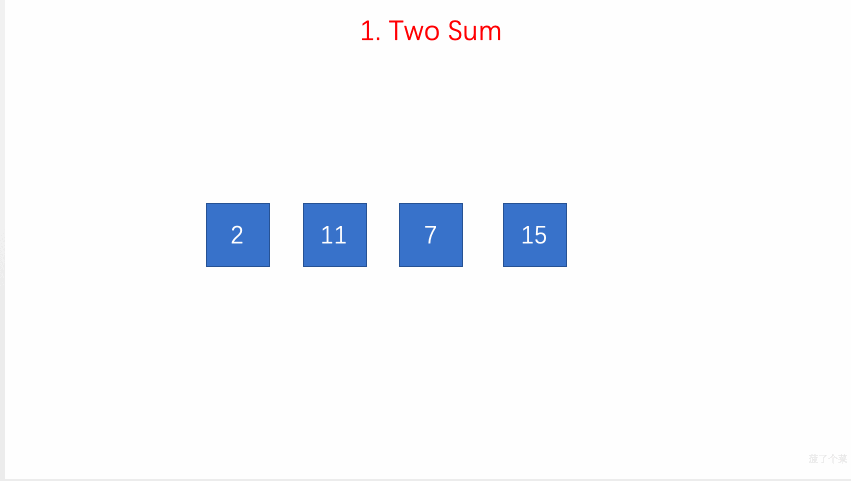# LeetCode 第 1 号问题：两数之和

### 题目描述

``````给定 nums = [2, 7, 11, 15], target = 9

``````

### 题目解析

• 每次遍历时使用临时变量 complement 用来保存目标值与当前值的差值
• 在此次遍历中查找 record ，查看是否有与 complement 一致的值，如果查找成功则返回查找值的索引值与当前变量的值 i
• 如果未找到，则在 record 保存该元素与索引值 i

### 动画描述### 代码实现

``````// 1. Two Sum
// https://leetcode.com/problems/two-sum/description/
// 时间复杂度：O(n)
// 空间复杂度：O(n)
class Solution {
public:
vector<int> twoSum(vector<int>& nums, int target) {
unordered_map<int,int> record;
for(int i = 0 ; i < nums.size() ; i ++){

int complement = target - nums[i];
if(record.find(complement) != record.end()){
int res[] = {i, record[complement]};
return vector<int>(res, res + 2);
}

record[nums[i]] = i;
}
}
};

``````